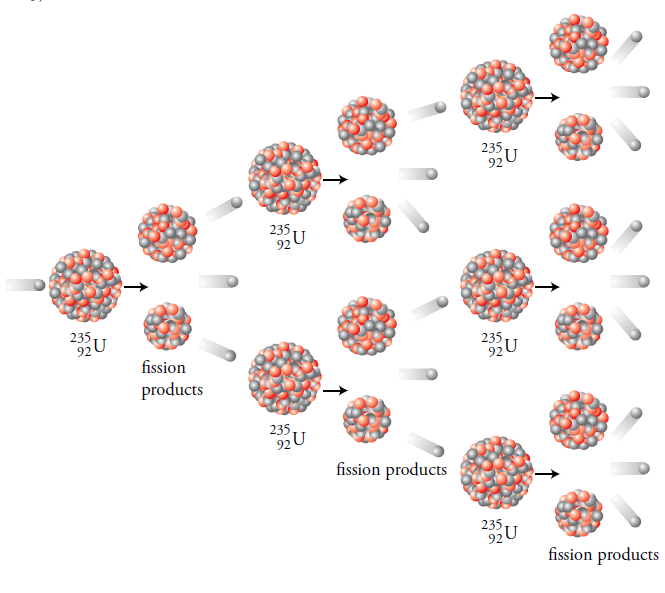## Thursday, October 6, 2011

### What is the reaction cross section for Helium-3 and boron-10 isotopes for thermal neutrons?

The reaction crossection for Helium-3 is 5330 Barns and that for Boron-10 is 3840 Barns.
Both the reactions are strongly  dependent on incident neutron energy 'E' and have roughly a 1/sqrt(E) dependence.

### Small trick for designers using capacitors

Suppose you require a smaller capacitance with higher voltage rating for your application but you are left with only higher capacitance capacitor with less voltage rating. How could you overcome this problem?

You can follow a small trick. You put two or more capacitors in series to arrive at your designed capacitance of smaller value.Because
i) It divides the voltage over two capacitors and thus has net effect of doubling (in case of two capacitors) the voltage rating.
ii) It reduces overall capacitance by following

C = (C1*C2) / (C1+C2 )

where 'C' is total capacitance of two capacitors in series.

For instance, consider two capacitors 100 pF @500VDC,

Then keeping them in series we get C = 50pF

voltage is now divided over two capacitors so total rating is 50pF @1000V Dc.

Hope this is helpful for designers.

### What is Decibel?

The human ear has logarithmic characteristics i.e. the loudness of sound is proportional to log of power which causes it.

Suppose a certain sound power 'P' produces a loudness 'L'. Now let loudness be increased to 'mL' by increasing power to 'kP'.(k, m are constants)

If loudness is again increased by same amount i.e 2mL, it is found that power required is K^2 * P.

If process is repeated 'N' times then

Pn = K^N * P  ---> log(Pn / P) =N*logK

N = [log(Pn/P)] / log K

taking natural log of above equation we get
N = K*log(Pn/P)

but napiers logs are difficult to work.

taking logs to base 10 we arrive at

N = 10 * log10(Pn/P)

where 'Pn' is power derived and 'P' is power absorbed.

The unit of above equation is decibel.

### Checking capacitors with an ohmmeter

Set the range of Ohm meter to higher range greater than 1MOhm. The ohm-meter leads are to be connected across leads of capacitor. Fora good capacitor, the meter first shows low value and then slowly recedes to a higher value. The reading gets stabilized to  a higher value after some time due to charging action.

For ceramic, paper and Mica capacitors, the resistance falls in the range 500 - 1000 MOhm.
For electrolytic capacitors, it is around 1MOhm.

If ohmmeter reading immediately goes to zero and stays there, the capacitor is short circuited.

If capacitor shows no charging action but just reads very high resistance it may be open.

Stray capacitance:

The wiring and components in a circuit have capacitance to metal chassis. The stray capacitance is typically 5 to 10PF.

Leakage resistance of capacitor:

Consider a capacitor charged by a DC voltage source. If the source is removed a perfect capacitor would keep its charge indefinitely.

## Saturday, September 24, 2011

### What is criticality in a Nuclear reactor?

Let us assume that a reactor is producing 1 Watt of power steadily as a result of 3.1 x 10^10 fissions per second.

The number of Neutrons available for fission at any time remains same during criticality condition. When the chain reaction is maintained steady, the power level is steady and the reactor is said to be critical.

If the power is increasing or decreasing, the rate of neutron production is not constant.

The neutron multiplication factor, K, based on the neutron cycle is used to keep track of neutron production.

K = number of neutrons in a generation / number of neutrons in preceding generation

For instance, consider 100 neutrons, which is our first generation.

If K=1, there will be 100 neutrons at beginning of second generation, 100 at third generation and so on. Fissions continue at the same rate as at the beginning.

If K>1, say 1.05, the 100 neutrons of first generation produce 100 x 1.05 = 105 neutrons at the beginning of next generation.

After 100 generations, the number of neutrons present would be 13150 i.e.100 x (1.05)^100.

The power would be increasing and is said to be super critical. In this case the power increased 131 times in about one tenth of a second. This is too fast to control and in practice multiplication factor is never allowed to become so large.

If K<1, the neutron population decreases with time and power decreases and reactor is said to be subcritical.